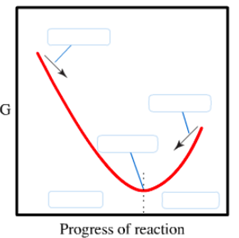# Problem: The diagram shows the free energy change of the reaction A(g) + B(g) ⇌ C(g).The reaction progress starts on the left with pure reactants, A and B each at 1 atm and moves to pure product, C, also at 1 atm on the right. Place the statements in the appropriate place on the diagram.spontaneousnonspontaneousQ&lt; KQ&gt; KQ= K

###### FREE Expert Solution
81% (198 ratings)
###### FREE Expert Solution

At Equilibrium:

Q (reaction quotient) = K (equilibrium constant)

81% (198 ratings)###### Problem Details

The diagram shows the free energy change of the reaction A(g) + B(g) ⇌ C(g).

The reaction progress starts on the left with pure reactants, A and B each at 1 atm and moves to pure product, C, also at 1 atm on the right. Place the statements in the appropriate place on the diagram.

spontaneous
nonspontaneous
Q< K
Q> K
Q= KWhat scientific concept do you need to know in order to solve this problem?

Our tutors have indicated that to solve this problem you will need to apply the The Reaction Quotient concept. You can view video lessons to learn The Reaction Quotient. Or if you need more The Reaction Quotient practice, you can also practice The Reaction Quotient practice problems.

What is the difficulty of this problem?

Our tutors rated the difficulty ofThe diagram shows the free energy change of the reaction A(g...as medium difficulty.

How long does this problem take to solve?

Our expert Chemistry tutor, Dasha took 2 minutes and 53 seconds to solve this problem. You can follow their steps in the video explanation above.

What professor is this problem relevant for?

Based on our data, we think this problem is relevant for Professor Jursich's class at UIC.